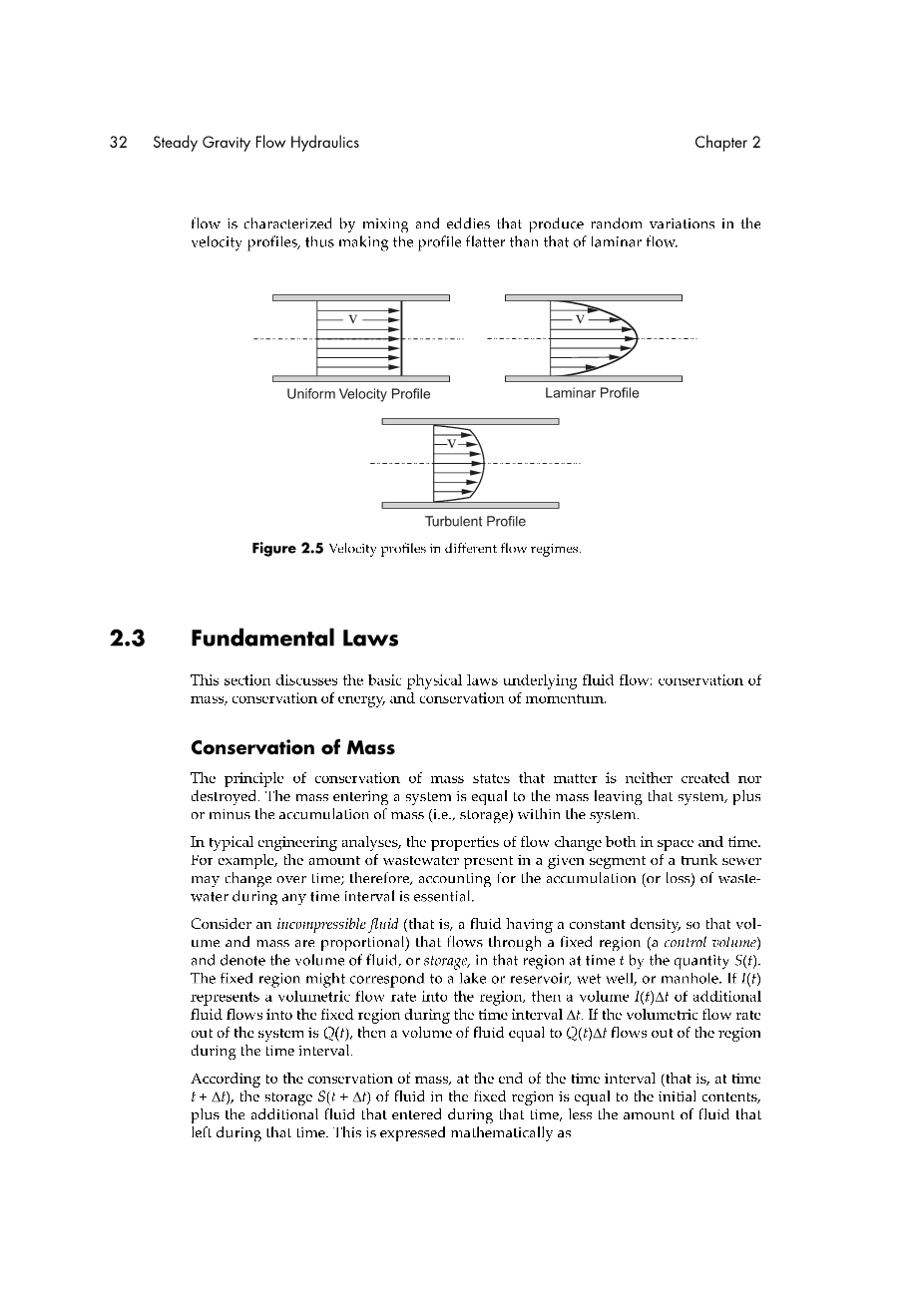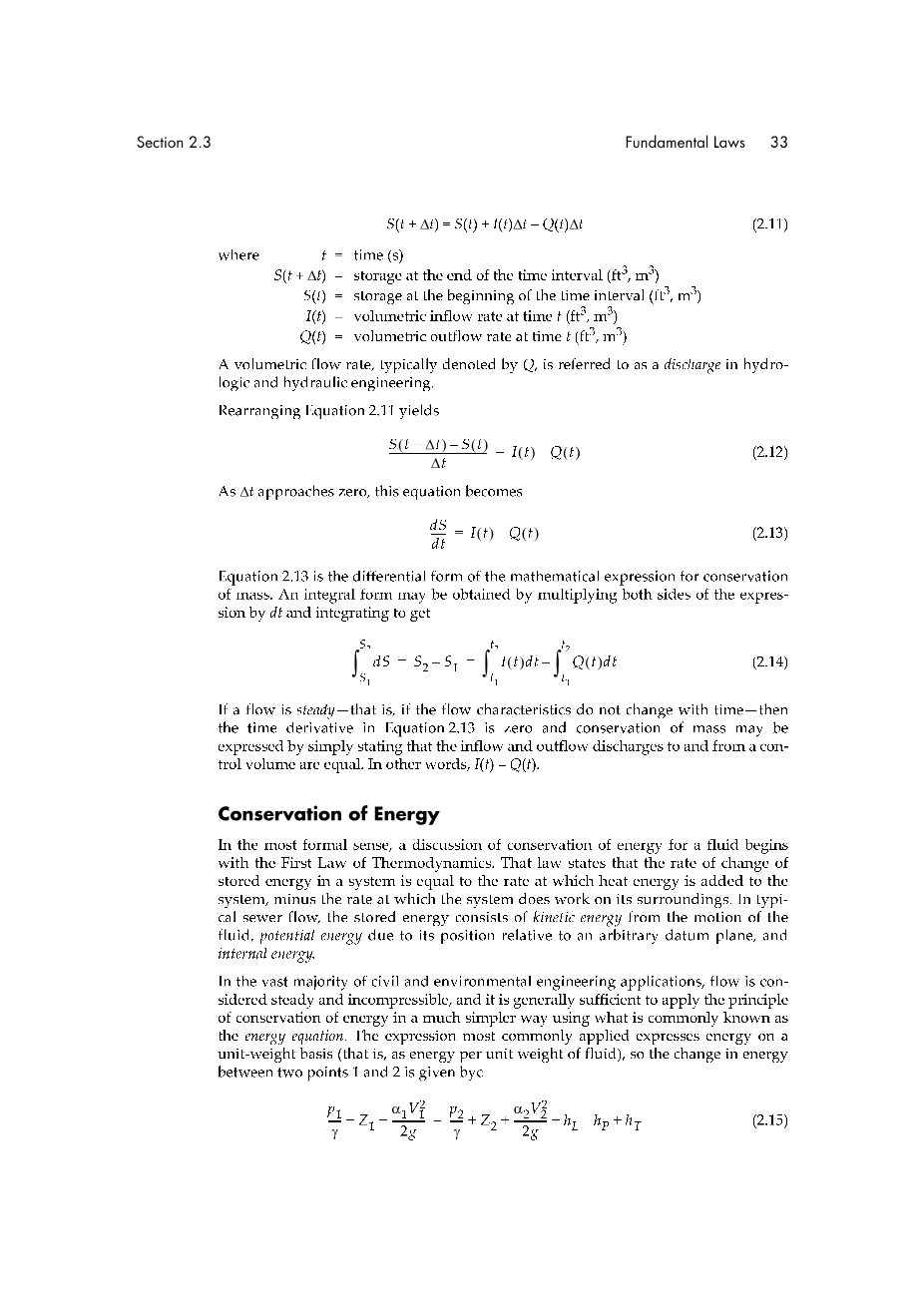# 紊流的特点是混合和涡流在速度剖面上产生随机波动，因此使速度分布比层流速度分布更加均匀外文翻译资料

2021-10-28 09:102.3 基本定律

S(t Delta;t）=时间间隔结束时的存量（ft3，m3

S（t）=时间间隔开始时的存量（ft3，m3

I（t）=时间t内的体积流入速率（ft3，m3

Q（t）=时间t内的体积流出速率（ft3，m3

gamma;=流体的重度（lb/ft3，N/m3)

Z=高于任意基准面的高度（ft，m）

alpha;=速度分布系数

V=流体速度，在横截面上平均（ft/s，m/s)

g=重力加速度常数（ft/s2，m/s2)

hL=横截面1和2之间的能量损失（ft，m）

hp=泵在横截面1和2之间提供的流体能量（ft，m）

hT=横截面1和2之间的涡轮损失的能量（ft，m）

d =流向通道底部的垂直深度(ft,m)

theta; =通道倾斜角

a =速度分布系数(无量纲)

V =流速(ft/s，m/s)

g=重力加速度常数(32.2 ft/s2，9.81 m/s2)

hL=第1节与第2节之间的能量损失(ft,m)

t =时间(s)

cv=控制体积(ft3, m3)

rho;=流体密度(slugs/ft3，kg/m3)

Vx=控制体积内流体速度的x分量(ft/s2，m/s2)

S =流体体积(ft3，m3)

Mout=控制体积的动量流出率(lb,N)

Mim =控制体积的动量流入率(lb,N)

beta; =速度分布系数(无量纲)

Q =流量(ft/s3，m/s3)

2.4 水力设计变量

2.5能量和水头损失